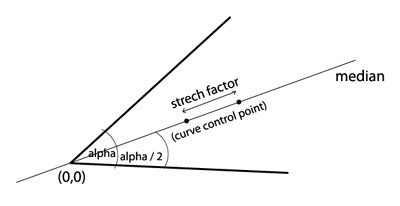Spiderweb class from last post has a major disadvantage because it uses central web point (0,0) as control point of every curve. This doesn't allow much flexibility, so I've figured how to correct it and make our web look like real life object. The solution is to introduce one new parameter - stretch factor.

Take a look at the image below. Stretch factor finds where on the angle median is control point located. This value actually multiplies x and y position of point on circle which intersects median with that circle. OK?If stretch factor is 1.0, spiderweb consist of concentric circles, if stretch factor is below 0.1, web will look much like examples from previous post and if value is greater than 1.0 you will get some flower-like shape. Values which mimics real life web are about 0.75 - 0.9 in my opinion, but you may find it different.

Here are some examples.When you call spiderweb you have 5 parameters now : new spiderweb(a, b, c, d, sf);

Spiderweb.as
`package com.blogspot.flanture.drawing{// Spiderweb drawing class// author: http://flanture.blogspot.com// version: 1.0// February 2010 import flash.display.Sprite; import flash.geom.Point;  public class spiderweb extends Sprite {  private var _circles:uint; // number of concentric circles  private var _nods:uint; // number of nods on each circle  private var _df:Number; // circles distance factor  private var _fr:Number; // smallest circle radius  private var _sf:Number; // stretch factor    public function spiderweb(circles:uint, nods:uint, fr:Number, df:Number, sf:Number)  {   _circles = circles;   _nods = nods;   _fr = fr;   _df = df;   _sf = sf;      init();  }    private function init():void  {   var cx = 0;   var cy = 0;   var coords:Array = new Array();   var controls:Array = new Array();   var angle:Number;   var controlAngle:Number;   var currR:Number;   var angleStep:Number = 360 / _nods;      currR = _fr;   for(var k:uint = 0; k < _circles; k++)   {    currR += currR * _df;        for (var i:uint = 0; i < _nods; i++)    {     angle = angleStep * (i + 1);     controlAngle = angle - angleStep / 2;          controlAngle = controlAngle * Math.PI / 180;     angle = angle * Math.PI / 180;          var x1:Number = trim(currR * Math.cos(angle));     var y1:Number = trim(currR * Math.sin(angle));          var tx:Number = trim(currR * Math.cos(controlAngle));     var ty:Number = trim(currR * Math.sin(controlAngle));          tx *= _sf;     ty *= _sf;          var cpoint:Point = new Point(tx, ty);     controls.push(cpoint);          var point:Point = new Point(x1, y1);     coords.push(point);         }        drawStraights(coords);    drawCurves(coords, controls);    coords.splice(0, _nods);    controls.splice(0, _nods);   }  }    private function trim(num:Number):Number  {   var nstr:int = int(num*100);   return Number(nstr / 100);  }    private function drawStraights(array):void  {   this.graphics.lineStyle(1, 0xffffff);   this.graphics.moveTo(0,0);   for (var i:uint = 0; i < array.length; i++)   {    this.graphics.lineTo(array[i].x, array[i].y);    this.graphics.moveTo(0,0);   }  }    private function drawCurves(arrayA, arrayB):void  {      this.graphics.lineStyle(2, 0xffffff);   for (var j:uint = 0; j < arrayA.length-1; j++)   {    this.graphics.moveTo(0,0);    this.graphics.moveTo(arrayA[j].x, arrayA[j].y);    this.graphics.curveTo(arrayB[j+1].x, arrayB[j+1].y, arrayA[j+1].x, arrayA[j+1].y);    this.graphics.moveTo(0,0);   }   this.graphics.moveTo(arrayA[arrayA.length-1].x, arrayA[arrayA.length-1].y);   this.graphics.curveTo(arrayB.x, arrayB.y, arrayA.x, arrayA.y);     } }}`

I hope you like the code.

*_*

1.What a coincidence! I just need such spiderweb to use in my project (commercial). On what license you publish that code?
Thanks for sharing ;)

2.code is under creative commons license, just link back to my blog and that's it

3.Hi, ur blog is really nice & informative, while reading it I truly like it. I just wanna suggest that u should submit your blog in this website which is offering very unique features at cheap prices there are expert advertising team who will not only provide the adspace but also promote ur blog & affiliate ads through all over the networks which will definitely boost ur traffic & readers. Finally I have bookmarked ur blog & also shared to my friends. hope u have a wonderful day & !!happy blogging!!.

Thanks for sharing your thoughts !